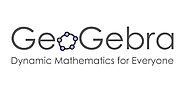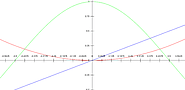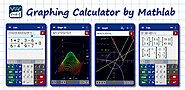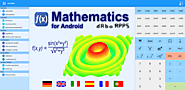Updated by Mertxe J. Badiola on Apr 22, 2019
REPORTMertxe J. Badiola
Owner
13 items   2 followers   0 votes   122 views

# Apps MATH

Apps para móviles y tabletas para utilizar en actividades del Área de Matemáticas

4

## GeoGebra 3D Graphing Calculator - Apps on Google PlayEasily solve 3D math problems, graph 3D functions and surfaces, create geometric constructions in 3D, save and share your results. Millions of people around the world use GeoGebra to learn mathematics and science. Join us: Dynamic Mathematics for everyone!• Plot f(x,y) functions and parametric surfaces• Create solids, spheres, planes and many more 3D objects• Get intersection points and cross-sections• Experience sliders, points, graphs and geometry all working together• Search for free learning activities directly from our app• Save and share your results with friends and teachersVisit www.geogebra.org to learn more and find all our free GeoGebra apps. We’d love to hear from you: send us your questions or feedback via Twitter @geogebra or to support@geogebra.org

2

## GeoGebra Classic - Apps on Google Play3

## GeoGebra Geometry - Apps on Google Play1

## GeoGebra Graphing Calculator - Apps on Google PlayEasily graph functions, solve equations, find special points of functions, save and share your results. Millions of people around the world use GeoGebra to learn math and science. Join us!• Plot functions, polar and parametric curves • Solve equations with our powerful math engine• Experience transformations with sliders• Get special points of functions: roots, min, max, intersections• Find derivatives and integrals• Do regression with best-fit lines • Search for free learning activities directly from our app• Save and share your results with friends and teachersVisit www.geogebra.org to learn more and find all our free GeoGebra apps. We’d love to hear from you: send us your questions or feedback via Twitter @geogebra or to support@geogebra.org

5

## Grapher - Apps on Google PlayPlot functions and trace their graphs with ease! This is a simple graphing calculator with graphing capabilities similar to TI-83 or TI-89 calculators. To start adding equations, tap the graph! There are no ads, because I hate ads.Features:* Graph tracer via trackball, trackpad, d-pad(hard or soft)** Press for current trace position** Up/down to change functions* Find intersection of 2 graphs* Save graph to SD card in "My Graphs"* Pinch-to-zoom and panning* Full screen graphing calculator* Custom equation keyboard* All colors are customizable* Long press to bring up soft d-pad (top or bottom)* Install on external mediaI would love to hear suggestions for any improvements or if you have problems using this program. Please email me if you think something is missing or if you would like to request functionality.I am not able to respond to comments, so please send an email so I can be clear on your request.(aka graph generator, function grapher, function plotter, math grapher, graphing calculator, function generator, maths graph)

14

## Graphing Calculator + Math, Algebra & Calculus - Apps on Google PlayGraphing Calculator by Mathlab is a scientific graphing calculator integrated with algebra and is an indispensable mathematical tool for students from high school to those in college or graduate school, or just anyone who needs more than what a basic calculator offers. It is designed to replace bulky and costly handheld graphing calculators and works on virtually any Android phone or tablet.

Video:
Help site with instructions and examples: http://help.mathlab.us
If you have a question, send email to calc@mathlab.us

SCIENTIFIC CALCULATOR
• Square root, cube and higher roots (hold √ key)
• Exponent or power, use x^ key, (x^2)
• Logarithms ln(), log(), logbase
• Trigonometric functions sin π/2, cos 30°, ...
• Hyperbolic functions sinh, cosh, tanh, ... (hold "e" key to switch)
• Inverse functions (hold direct function key)
• Complex numbers, all functions support complex arguments
• Derivatives sin x' = cos x, ... (hold x^ key)
• Scientific and engineering notation (enable in menu)
• Percent mode
• Binary, octal and hexadecimal numbers, 0b1010, 0o123, 0xABC

GRAPHING CALCULATOR
• Multiple functions graphing
• Implicit functions up to 2nd degree (ellipse 2x^2+3y^2=1, etc.)
• Polar graphs (r=cos2θ)
• Parametric functions, enter each on new line (x=cos t, y=sin t)
• Function roots and critical points.
• Graph intersections
• Tracing function values and slopes
• Slide to scroll
• Pinch to zoom
• Fullscreen graphs (PRO)
• Function tables
• Save graphs as images
• Save tables as csv
• 3D graphs (PRO)

FRACTION CALCULATOR
• Simple and complex fractions 1/2 + 1/3 = 5/6
• Mixed numbers, use space to enter values 3 1/2
• Use parentheses (1+2)/(3+4)=3/7

ALGEBRA CALCULATOR
• Linear equations x+1=2 -> x=1
• Quadratic equations x^2-1=0 -> x=-1,1
• Approximate roots of higher polynomials
• Systems of linear equations, write one equation per line, x1+x2=1, x1-x2=2
• Polynomial long division
• Polynomial expansion, factoring

PROBABILITY AND STATISTICS
• Hold n! key to enter functions, double tap dot to enter comma
• Combinations nCr(5,2)=10
• Permutations nPr(5,2)=20
• Sample A=[1 2 3 4 5]
• Sample B=[2 3 4 5 6]
• Sum sum(A) = 15
• Average avg(A) = 3
• Median median(A) = 3
• Sample variance var(A) = 2.5
• Population variance varp(A) = 2
• Standard deviation stdev(A) = 1.58..., stdevp(A) = 1.41...
• Covariance cov(A,B) = 2.5
• Correlation corr(A,B) = 1

MATRIX CALCULATOR
• Matrix and vector arithmetic operations
• Vector cross product, dot product (hold *) and norm
• Matrix determinant, inverse, transpose and trace functions

LIBRARY
• Physical constants (PRO)
• User defined constants and functions (PRO)
• Save expressions for future reference

6

## Graphing Calculator + Math, Algebra & Calculus - Apps on Google PlayGraphing Calculator by Mathlab is a scientific graphing calculator integrated with algebra and is an indispensable mathematical tool for students from high school to those in college or graduate school, or just anyone who needs more than what a basic calculator offers. It is designed to replace bulky and costly handheld graphing calculators and works on virtually any Android phone or tablet.Free version requires internet connection and contains ads! Upgrade to PRO!Video: https://youtu.be/6BR8Lv1U9kAHelp site with instructions and examples: http://help.mathlab.usIf you have a question, send email to calc@mathlab.usSCIENTIFIC CALCULATOR• Square root, cube and higher roots (hold √ key)• Exponent or power, use x^ key, (x^2)• Logarithms ln(), log(), logbase• Trigonometric functions sin π/2, cos 30°, ...• Hyperbolic functions sinh, cosh, tanh, ... (hold "e" key to switch)• Inverse functions (hold direct function key)• Complex numbers, all functions support complex arguments• Derivatives sin x' = cos x, ... (hold x^ key)• Scientific and engineering notation (enable in menu)• Percent mode• Binary, octal and hexadecimal numbers, 0b1010, 0o123, 0xABC• Save and load historyGRAPHING CALCULATOR• Multiple functions graphing• Implicit functions up to 2nd degree (ellipse 2x^2+3y^2=1, etc.)• Polar graphs (r=cos2θ)• Parametric functions, enter each on new line (x=cos t, y=sin t)• Function roots and critical points.• Graph intersections• Tracing function values and slopes• Slide to scroll• Pinch to zoom• Fullscreen graphs (PRO)• Function tables• Save graphs as images• Save tables as csv• 3D graphs (PRO)FRACTION CALCULATOR• Simple and complex fractions 1/2 + 1/3 = 5/6• Mixed numbers, use space to enter values 3 1/2• Use parentheses (1+2)/(3+4)=3/7 ALGEBRA CALCULATOR• Linear equations x+1=2 -> x=1• Quadratic equations x^2-1=0 -> x=-1,1• Approximate roots of higher polynomials• Systems of linear equations, write one equation per line, x1+x2=1, x1-x2=2• Polynomial long division• Polynomial expansion, factoring• Linear and quadratic inequalitiesPROBABILITY AND STATISTICS• Hold n! key to enter functions, double tap dot to enter comma• Combinations nCr(5,2)=10• Permutations nPr(5,2)=20• Sample A=[1 2 3 4 5]• Sample B=[2 3 4 5 6]• Sum sum(A) = 15• Average avg(A) = 3• Median median(A) = 3• Sample variance var(A) = 2.5• Population variance varp(A) = 2• Standard deviation stdev(A) = 1.58..., stdevp(A) = 1.41...• Covariance cov(A,B) = 2.5• Correlation corr(A,B) = 1 MATRIX CALCULATOR• Matrix and vector arithmetic operations• Vector cross product, dot product (hold *) and norm• Matrix determinant, inverse, transpose and trace functionsLIBRARY• Physical constants (PRO)• User defined constants and functions (PRO)• Save expressions for future reference

10

## Matemáticas Divertidas - Apps on Google PlayÉste juego te permite jugar y aprender matemáticas al mismo tiempo, permitiéndote jugar con operaciones aritméticas, con punto decimal y fracciones. El principio está basado de Tuxmath pero agregando nueva funcionalidad y formas de juego.Además puedes jugar en red local con algún rival y probar tus conocimientos matemáticos. Es ideal para cualquiera que requiera practicar operaciones matemáticas y aprender jugando al mismo tiempo.

7

## Mathematics - Apps on Google Play• formula calculation with variables, sums, products and sequences- solve equations (linear, quadratic, cubic, transpose)- plot functions, derivate, integrate, calculate roots and extremas (polynomial, rational, exponential, etc)- calculate tangent, asymptote, interception- reconstruction: calculate f(x) by given roots, points or extremas- f(x,y) plot in 3D- factoring (Bézout's identity, Euler's totient function, least common multiple, greatest common divisor)- modulo calculation with big integer and fractions- differential and integral calculus- curve sketching, limes, minima, maxima- linear algebra, vectors, matrices- unit conversion- number systems, binary/oct/decimal/hex calculator- complex number calculation- statistics (gaussian test, t test, binominal and normal distribution, standard deviation, average, sum, median)Supported languages:English, German, Francais, Espanol, Italian, PortugueseMathematics is a powerful calculation software for your android smartphone.FEATURESCalculate any formula you want and show them in a 2d or 3d plot. The natural display shows fractions, roots and exponents as you would expect it from mathematics.In a few seconds you derivate or integrate your desired function, calculate the zero points of your function und show them in the function plot. See all maxima, minima or inflection points in one view.The easy way of use allows you to solve linear equations in just a moment. Or transform your mathematical, physical or chemical equation to any unknown variable.You often needs to calculate with binary, octal or hexadecimal number systems? No problem! You can mix them together in one calculation even by using decimal digits. But that's not enough! You can also calculate with any other number system with base 2 to 18.From time to time you may need to convert units to another one, like Celsius to Fahrenheit, miles to kilometre, inches to foot and so on.You will also be able to calculate with vectors, matrices and determinants.All this features are combined in this app and will make your mathematical life a lot easier.
8

## Mathway - Apps on Google Play9

## MATLAB Mobile - Apps on Google Play13

## Photomath - Apps on Google PlayLearn how to solve math problems, check homework assignments and study for upcoming exams and ACTs/SATs with the world’s most used math learning resource. Over 100+ million downloads, and billions of problems solved every month! Photomath is FREE and works without wi-fi.

HOW IT WORKS
Instantly scan printed text AND handwritten math problems using your device’s camera or type and edit equations with our scientific calculator. Photomath breaks down every math problem into simple, easy-to-understand steps so you can really understand core concepts and can answer things confidently.

KEY FEATURES
Scan textbook (print) AND handwritten problems
Scientific calculator
Step-by-step explanations for every solution
Multiple solving methods
No internet connection required to use
30+ languages supported
Interactive graphs

MATH TOPICS
Basic Math/Pre-Algebra: arithmetic, integers, fractions, decimal numbers, powers, roots, factors
Algebra: linear equations/inequalities, quadratic equations, systems of equations, logarithms, functions, matrices, graphing, polynomials
Trigonometry/Precalculus: identities, conic sections, vectors, matrices, complex numbers, sequences and series, logarithmic functions
Calculus: limits, derivatives, integrals, curve sketching
Statistics: combinations, factorials

Our in-house team of veteran math educators also partner with teachers worldwide to ensure we’re utilizing the most effective, contemporary teacher methodologies in our math engines.

Featured in Huffington Post, Forbes, TIME, CNN, EdSurge, Guiding Tech, The Verge, TechCrunch and more.

Suggestions, comments or questions? Email us at support@photomath.net

Photomath is and will always be free to use, but you can boost your learning by upgrading to Photomath Plus. Photomath Plus provides solutions to full textbooks and problem sets with word problems! Currently restricted to the US and specific textbooks only.

Payment will be charged to your Google Play account at the confirmation of purchase. Subscription automatically renews unless it is canceled at least 24 hours before the end of the current period. Your account will be charged for renewal within 24 hours prior to the end of the current period. You can manage and cancel your subscriptions by going to your account settings on the Google Play after purchase. Offers and pricing subject to change without notice.